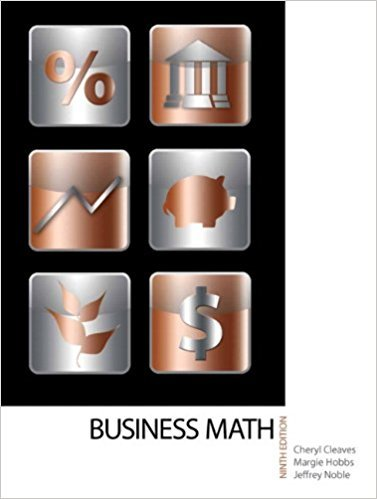×
×

# Complete the tables for Exercises 910. Round to the nearest tenth. 8000ISBN: 9780135108178 355

## Solution for problem 10 Chapter 18

• Textbook Solutions
• 2901 Step-by-step solutions solved by professors and subject experts
• Get 24/7 help from StudySoup virtual teaching assistants4 5 1 415 Reviews
30
2
Problem 10

Complete the tables for Exercises 910. Round to the nearest tenth. 8000

Step-by-Step Solution:
Step 1 of 3

tr 1-' -''"trlg e I \+g- l n \It\t V Ii'-r i '- \*.'t, *"#h*(-\^ x+- -=t\^ -+-+4/ V -V)r +- - -T (t- \)!- - G' nV) \L 'l-i"-...

Step 2 of 3

Step 3 of 3

##### ISBN: 9780135108178

The answer to “Complete the tables for Exercises 910. Round to the nearest tenth. 8000” is broken down into a number of easy to follow steps, and 12 words. Since the solution to 10 from 18 chapter was answered, more than 232 students have viewed the full step-by-step answer. The full step-by-step solution to problem: 10 from chapter: 18 was answered by , our top Math solution expert on 03/08/18, 08:36PM. This full solution covers the following key subjects: . This expansive textbook survival guide covers 77 chapters, and 1559 solutions. Business Math, was written by and is associated to the ISBN: 9780135108178. This textbook survival guide was created for the textbook: Business Math, , edition: 9.

Unlock Textbook Solution